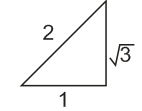Q

# How to solve this problem- - Co-ordinate geometry - JEE Main-10

If the normal to the ellipse  at a point P on its parallel to the line,  and the tangent to the ellipse at P passes through   then PQ is equal to :

• Option 1)

• Option 2)

• Option 3)

• Option 4)

Views

So,

Let

Equation of normal is

Equation of tangent is it passes throughHence point is

Option 1)

Option 2)

Option 3)

Option 4)

Exams
Articles
Questions